• 2021-10-12 10:05:20

## 方法1：向量叉乘判别法

(非凹多边形，凹多边形需要切割为凸多边形)

设多边形的顶点依次为A1，A2…An，要判断的点为P，那么分别计算向量PA1叉乘向量PA2，向量PA2叉乘向量PA3，…，向量PA(n-1)叉乘向量PAn，向量PAn叉乘向量PA1，如果这些叉乘的结果都同向的话，那么这个点就在多边形的内部。

## 方法2：面积和判别法

判断目标点与多边形的每条边组成的三角形面积和是否等于该多边形，相等则在多边形内部。

### 具体做法：

设要检测的点为P点。用P点连接多边形各顶点，假如P点在多边形以内，则顶点与P组成的三角形正好填充此多边形。反之，则不能。此时使用多边形面积计算公式，使P点作为参考点（a,b,c,.......,n为多边形定点）则有：

S = 0.5 * ( (a.x-p.x)*(a.y-p.y)-(b.x-p.x)*(b.y-p.y) +(b.x-p.x)*(b.y-p.y)-(c.x-p.x)*(c.y-p.y) +......... + (n.x-p.x)*(n.y-p.y)-(a.x-p.x)*(a.y-p.y) )

如果P点不在多边形以内，则每个三角形的相对面积符号不会一致。由于0算法
更多相关内容
• 针对正多边形渐变为圆的算法进行了研究,提出了两种算法:加边法,即通过增加正多边形边数的方法逐渐逼近于圆,当正多边形的边数足够多时,可以认为其是一个圆;倒角法,逐渐增加正多边的倒角半径,当倒角半径趋近等于正...
• 通过对HTD 2M同步带轮的合理修形,减小带传动的多边形效应,提高带传动精度,并通过检测设备加以验证。研究表明:同步带嵌在齿槽的约1/2直线节距还原成1/2弧线节距后,Y轴所需要的变形量是0.051 2 mm,通过有限元计算的...
• 判断一个点是否在多边形内部的方法 面积和判别法：判断目标点与多边形的每条边组成的三角形面积和是否等于该多边形，相等则在多边形内部。 夹角和判别法：判断目标点与所有边的夹角和是否为360度，为360度则在...

## 判断一个点是否在多边形内部的方法

• 面积和判别法：判断目标点与多边形的每条边组成的三角形面积和是否等于该多边形，相等则在多边形内部。
• 夹角和判别法：判断目标点与所有边的夹角和是否为360度，为360度则在多边形内部。
• 引射线法：从目标点出发引一条射线，看这条射线和多边形所有边的交点数目。如果有奇数个交点，则说明在内部，如果有偶数个交点，则说明在外部

## 首先讲解下射线法的原理

### 情况一，显示了具有 14 条边的严重凹陷多边形的典型情况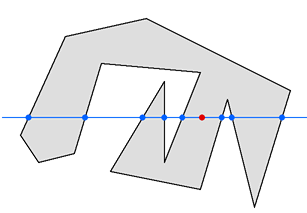• 上图 显示了具有 14 条边的严重凹陷多边形的典型情况

• 红点是需要测试的点，以确定它是否位于多边形内。解决方案是将多边形的每一侧与测试点的Y（垂直）坐标进行比较，并编译节点列表，其中每个节点都是一条线超过测试点的Y阈值的点。

• 在此示例中，多边形的八条边穿过 Y 阈值，而其他六条边则不然。然后，如果测试点的每一侧都有奇数个节点，则它位于多边形内部;如果测试点的每一侧都有偶数个节点，则它位于多边形之外。在我们的示例中，测试点的左侧有五个节点，右侧有三个节点。由于 5 和 3 是奇数，因此我们的测试点位于多边形内部。
（注意：此算法不关心多边形是以顺时针还是逆时针方式
跟踪.

### 情况二，显示了多边形自身交叉时发生的情况。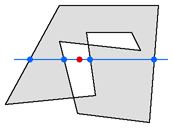在此示例中，十边形的线彼此相交。这种效果很像"独占或"或异或异或，因为汇编语言程序员都知道它。多边形中重叠的部分相互抵消。因此，测试点位于多边形之外，如其两侧的偶数个节点（两个和两个）所示。

### 情况三，六边形本身不重叠，但它确实有交叉的线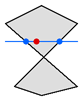这不是问题;算法仍然工作正常。

### 情况四，显示了多边形的情况，其中一条边完全位于阈值上。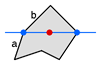既然边a和边 b都触及阈值，那么它们是否应该同时生成一个节点？不，因为这样测试点的每一侧都会有两个节点，因此测试会说它在多边形之外，而显然不是！

必须将恰好位于 Y 阈值上的点视为属于阈值的一侧。假设我们任意决定 Y 阈值上的点将属于阈值的"高于"一侧。然后，端a生成一个节点，因为它有一个终结点低于阈值，而另一个终结点位于或高于阈值。端b不会生成节点，因为它的两个端点都位于或高于阈值，因此它不被视为跨阈值端。

### 情况五，显示了多边形的情况，其中一条边完全位于阈值上。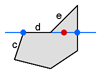只需遵循有关情况四所述的规则即可。端c生成一个节点，因为它有一个终结点低于阈值，而其另一个终结点位于阈值或阈值之上。端d不会生成节点，因为它的两个终结点都位于阈值上或之上。而端e也不会生成节点，因为它的两个端点都位于阈值上或之上。

### 情况六，一个特殊情况。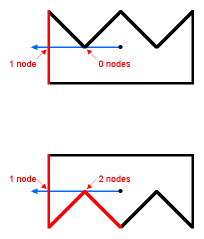• 多边形的一个内部角度正好触及测试点的 Y 阈值。这没关系。在上图中，只有一侧（以红色隐藏）在测试点的左侧生成一个节点，而在底部的示例中，三条边可以。无论哪种方式，该数字都是奇数，并且测试点将被视为在多边形内。

• 多边形边缘
如果测试点位于多边形的边界上，则此算法将提供不可预知的结果;即，结果可能是"内部"或"外部"，具体取决于任意因素，例如多边形相对于坐标系的方向。（这通常不是问题，因为多边形的边缘无论如何都是无限薄的，并且落在边缘上的点可以向任何方向移动而不会损害多边形的外观

### 直线表达式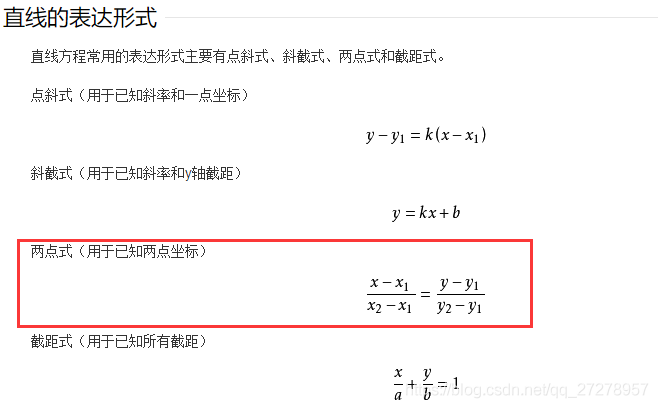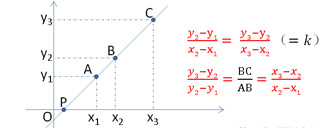• 我们可以水平/垂直去做射线，容易理解。

### 代码示例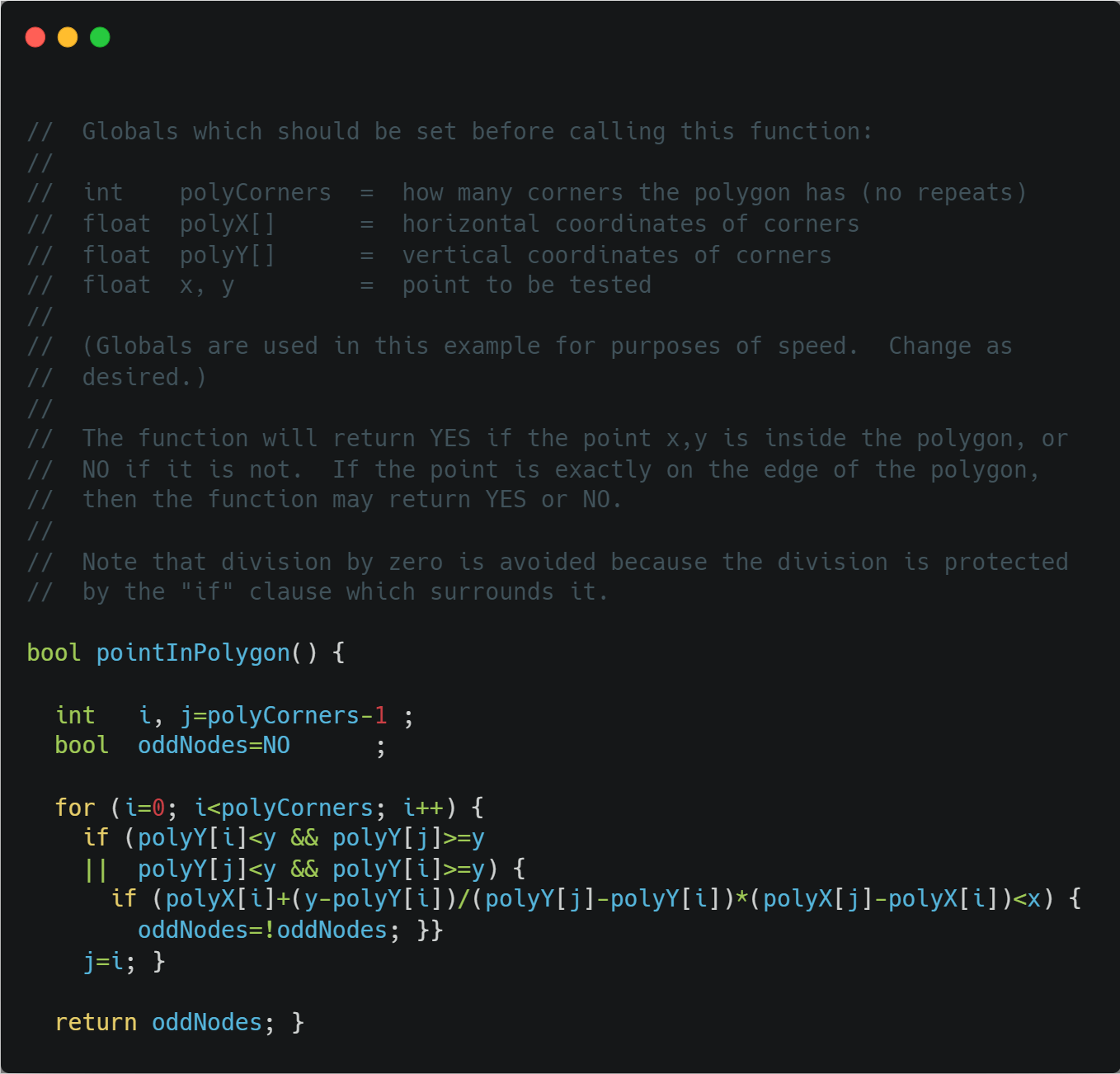• 这是Nathan Mercer提供的效率改进。紫色代码消除了完全位于测试点右侧的边上的计算。虽然对于某些多边形，这可能偶尔会变慢，但对于大多数多边形来说，这可能更快。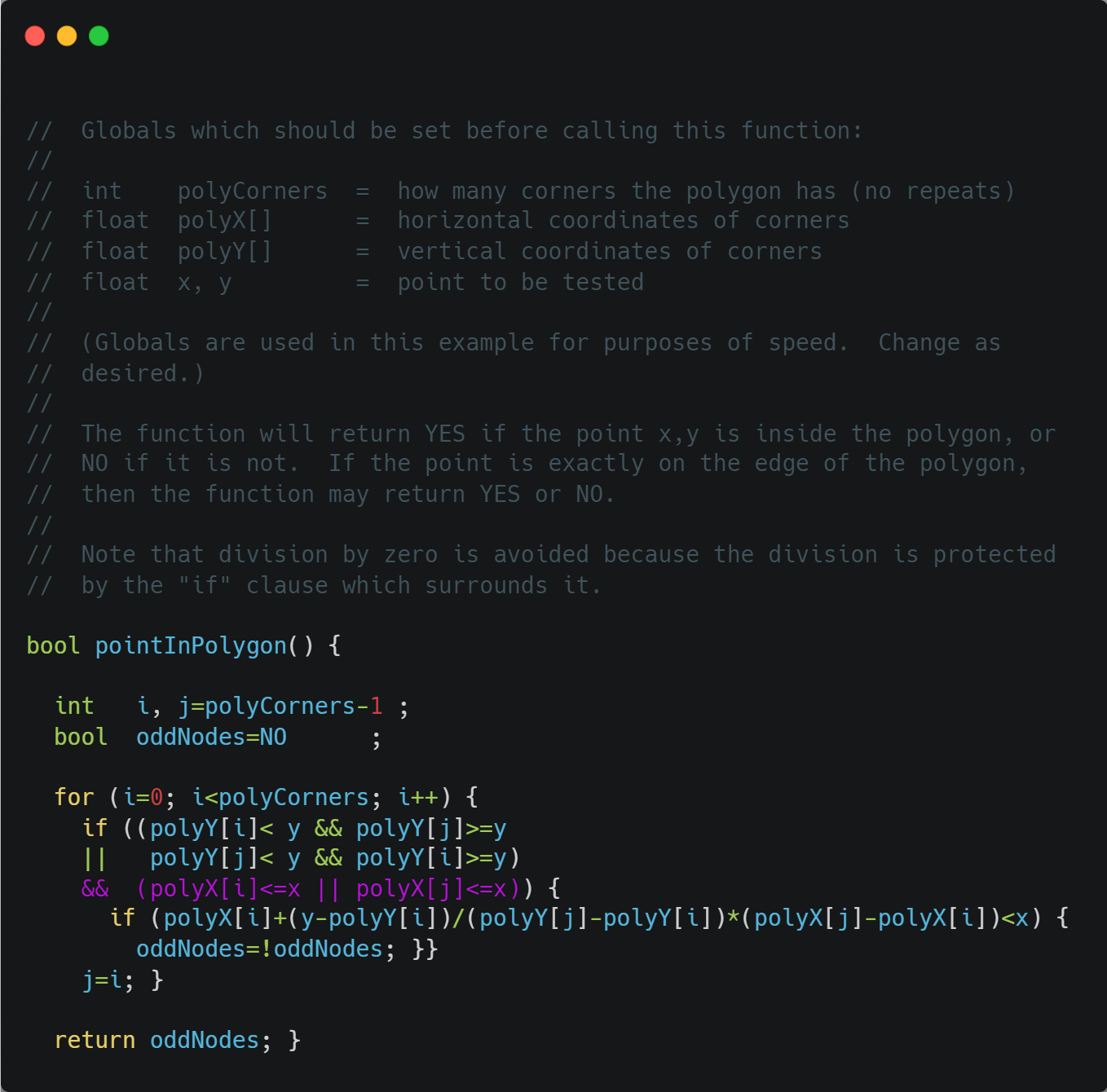• 另一个效率改进。内部的"if"语句被删除，取而代之的是独占 OR 操作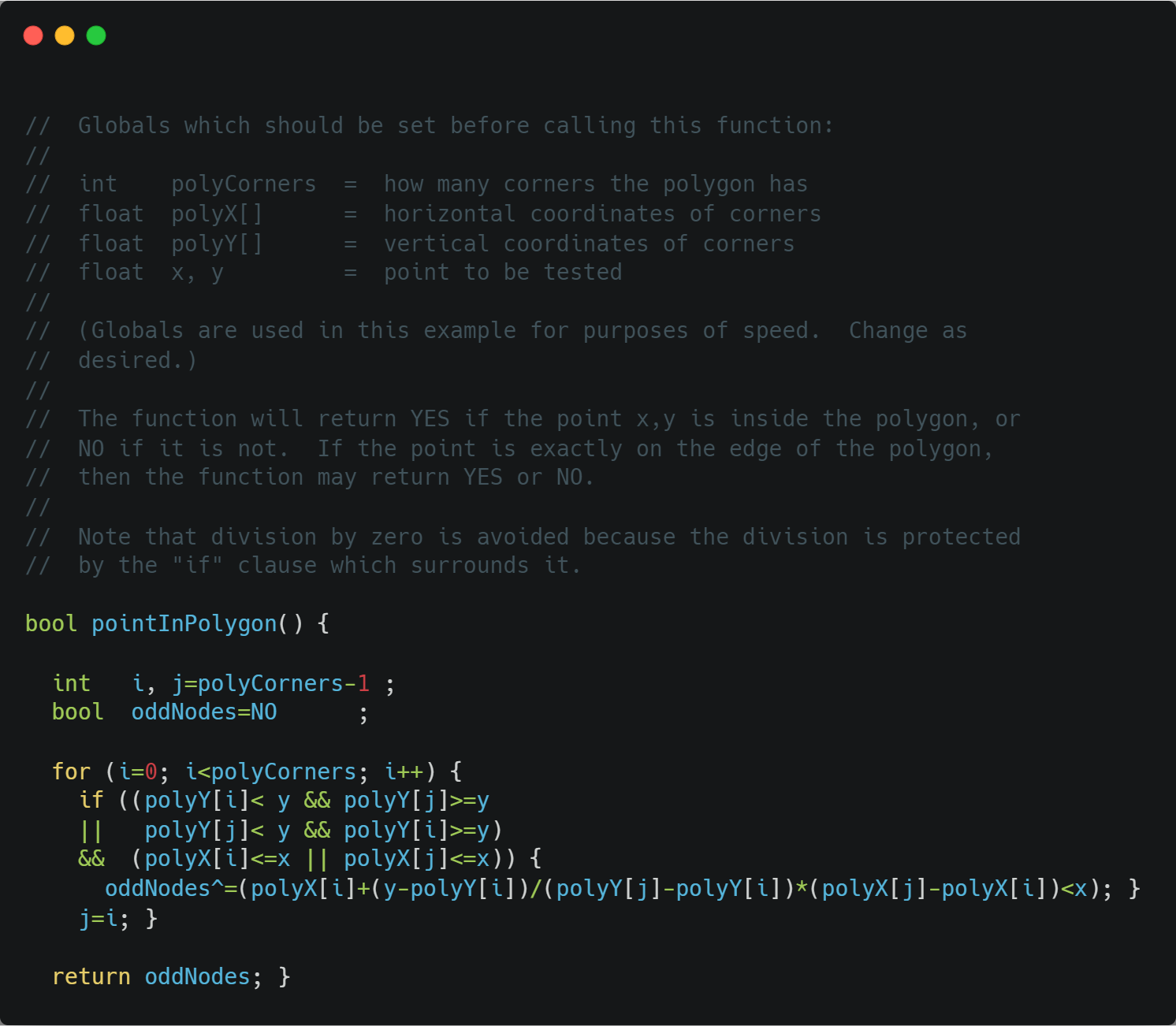• 如果您有许多点需要针对同一（静态）多边形进行测试，这将非常有用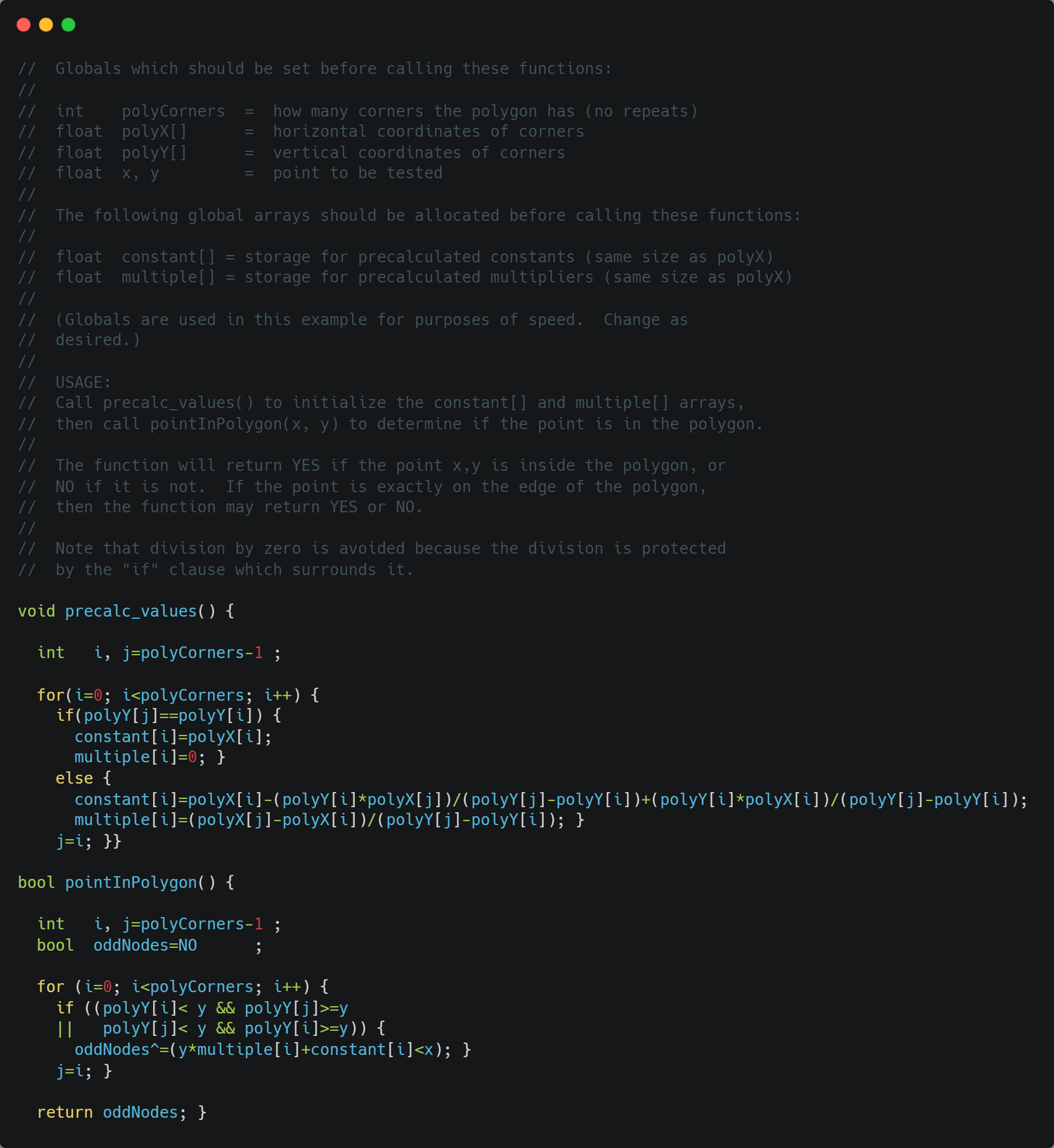• 个非常聪明的优化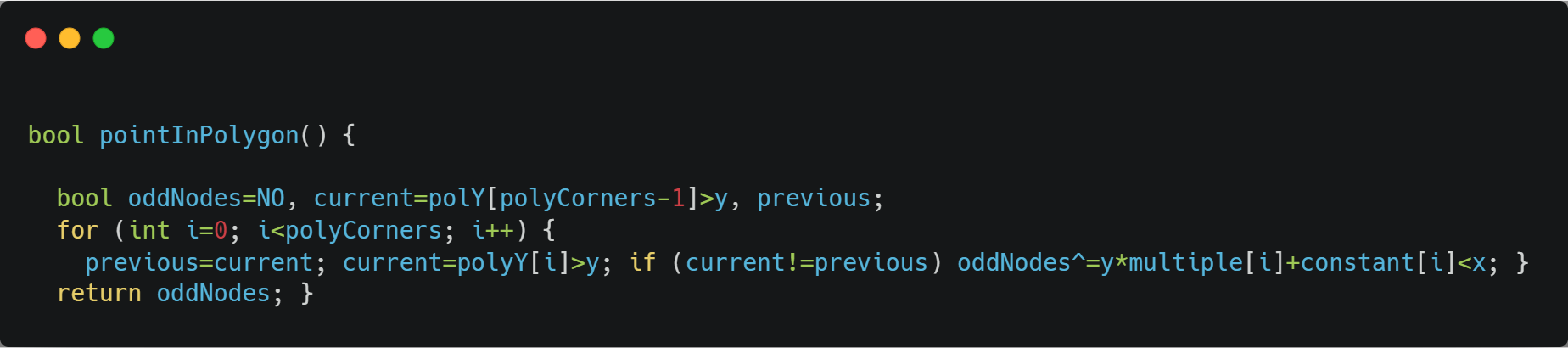## 一个C++ 示例代码## 参考连接

射线法查找点是否在多边形内

展开全文• 判定检测点是否在多边形内的新方法，陈树强，陈学工，本文提出一种新方法以检测一个点是否在多边形内。该方法将矢量和射线法结合，彻底解决了射线法所具有的奇异情况。实验结果证明
• 前言大家都知道MongoDB是一个...1.使用百度拾取坐标工具，在地图上定义多边形的坐标点，并把每个点的坐标保存。百度拾取坐标工具：http://api.map.baidu.com/lbsapi/getpoint/多边形的坐标点如下：113.314882,23.163...

前言

大家都知道MongoDB是一个基于分布式文件存储的数据库，并提供创建基于地理空间的索引的能力，本文将使用MongoDB 基于地理空间索引进行坐标所在区域的判断及使用。

1.使用百度拾取坐标工具，在地图上定义多边形的坐标点，并把每个点的坐标保存。

百度拾取坐标工具：http://api.map.baidu.com/lbsapi/getpoint/

多边形的坐标点如下：

113.314882,23.163055

113.355845,23.167042

113.370289,23.149564

113.356779,23.129758

113.338238,23.13913

113.330979,23.124706

113.313588,23.140858

113.323865,23.158204

113.314882,23.163055

注意：首尾坐标必须一样，这样才能使多边形闭合。

2.使用百度地图开放平台地图JS Demo，把多边形坐标输入，看看多边形是否合适。

百度地图开放平台地图JS Demo：http://developer.baidu.com/map/jsdemo.htm#c2_9

把以下代码替换源码编辑器中的内容，然后点击运行

body, html{width: 100%;height: 100%;margin:0;font-family:"微软雅黑";}

#allmap {height:100%; width: 100%;}

#control{width:100%;}

设置线、面可编辑

开启线、面编辑功能

关闭线、面编辑功能

// 百度地图API功能

var map = new BMap.Map("allmap");

map.centerAndZoom(new BMap.Point(113.330764,23.155878), 15);

map.enableScrollWheelZoom();

var polygon = new BMap.Polygon([

new BMap.Point(113.314882,23.163055),

new BMap.Point(113.355845,23.167042),

new BMap.Point(113.370289,23.149564),

new BMap.Point(113.356779,23.129758),

new BMap.Point(113.338238,23.13913),

new BMap.Point(113.330979,23.124706),

new BMap.Point(113.313588,23.140858),

new BMap.Point(113.323865,23.158204)

], {strokeColor:"blue", strokeWeight:2, strokeOpacity:0.5}); //创建多边形

多边形区域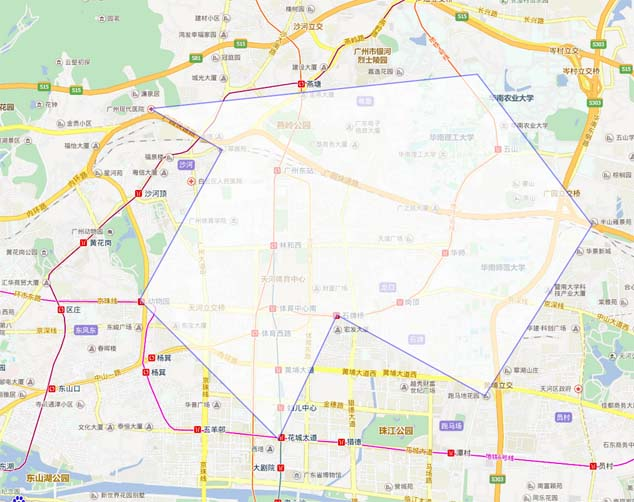3.定义测试坐标

广州东站坐标：113.330908,23.155678(多边形内)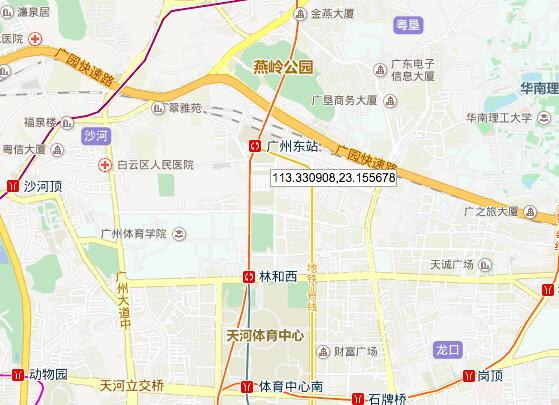宏发大厦：113.33831,23.137335(多边形外)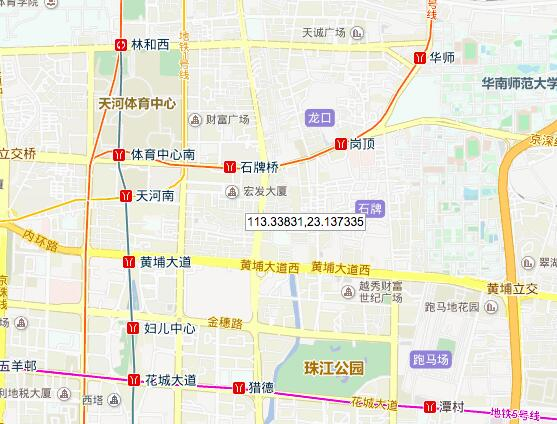4.在mongodb测试

1.创建数据库

use testdb;

db.createUser(

{

"user":"root",

"pwd":"123456",

}

);

db.auth(

{

"user":"root",

"pwd":"123456"

}

);

2.插入多边形范围并添加索引

db.geo.ensureIndex(

{

polygons: "2dsphere"

}

);

db.geo.insert(

{

polygons:

{

type:"Polygon",

coordinates:[[

[113.314882,23.163055],

[113.355845,23.167042],

[113.370289,23.149564],

[113.356779,23.129758],

[113.338238,23.13913],

[113.330979,23.124706],

[113.313588,23.140858],

[113.323865,23.158204],

[113.314882,23.163055],

]]

}

}

);

3.判断坐标是否在多边形区域

广州东站坐标：113.330908,23.155678

db.geo.find(

{

polygons:

{

$geoIntersects: {$geometry:{

"type" : "Point",

"coordinates" : [113.330908,23.155678] }

}

}

}

);

输出：

{ "_id" : ObjectId("57c2b1895fb7fd4790f9f099"), "polygons" : { "type" : "Polygon", "coordinates" : [ [ [ 113.314882, 23.163055 ], [ 113.355845, 23.167042 ], [ 113.370289, 23.149564 ], [ 113.356779, 23.129758 ], [ 113.338238, 23.13913 ], [ 113.330979, 23.124706 ], [ 113.313588, 23.140858 ], [ 113.323865, 23.158204 ], [ 113.314882, 23.163055 ] ] ] } }

表示坐标 113.330908,23.155678在多边形区域内

宏发大厦：113.33831,23.137335

db.geo.find(

{

polygons:

{

$geoIntersects: {$geometry:{

"type" : "Point",

"coordinates" : [113.33831,23.137335] }

}

}

}

);

输出：空

表示坐标 113.33831,23.137335在多边形区域外

总结

以上就是利用mongodb判断坐标是否在指定多边形区域内的全部内容了，希望本文的内容对大家的学习或者工作能带来一定的帮助，如果有疑问大家可以留言交流，谢谢大家对脚本之家的支持。

展开全文• 针对凸多边形的最小面积四边形包围盒问题进行研究，通过数学推导证明，得出了凸多边形的最小面积四边形包围盒的四边都是多共点边，或三边是多共点边而另一边（单共点边）中点与凸多边形的一顶点重合等一系列结论。...
• 多边形内外点判定算法是图形...最后进行实验仿真，证明该算法的平均时间复杂度为O（n/2）。该算法通过递归的分割凸多边形，判断待测点与分割线的相对位置，最终转化为三角形内外点的判别，具有快速、稳定、准确的优势。
• 方法首先针对三个时空维度和四个时空维度的单场情况开发，并在经典势能示例以及量子涨落的计算上得到了证明。 考虑到更高阶的校正，系统考虑了超出线性阶的电位的系统扩展，这为多个标量场铺平了道路。 因此，...
• 点在多边形内判断方法matlab实现（点在凸包内、任意形状内判定） 主要包含： 1 射线交叉点法 （Crossing Number） 2 环绕数法（Winding Number） 3 角度法（转角法） 3.1 角度相加法 3.2 改进角度法（matlab自带in...

### 点在多边形内判断方法matlab实现（点在凸包内、任意形状内判定）

惯例声明：本人没有相关的工程应用经验，只是纯粹对相关算法感兴趣才写此博客。所以如果有错误，欢迎在评论区指正，不胜感激。本文主要关注于算法的实现，对于实际应用等问题本人没有任何经验，所以也不再涉及。

# 0 前言

本文大概说一下目前常用计算机判断点在多边形内的方法。这个实际应用还是比较普遍的，比如单纯的几何意义上的判断，或者选定一个范围，看看数据是否超出这个范围之类的。

咱们人在判断点是否在多边形内，通常是先识别多边形图形，然后看看点是否在这个识别的图形范围内。但是对于很复杂的图形，就不容易一下子得到结论，而且这种基于图形的算法实际上效率并不高，不适用于计算机计算。

常用的判断点是否在多边形内的算法，与多边形的变数n相关，通常都是O(n)量级的，所以其实大家都相差不大，就看谁更能优化。但是对于凸多边形来说，可以用二分法算法，将计算复杂度降到O(logn)量级，这个在后面会涉及到。

之后如果有空，准备试一试写个判断点是否在三维以及高维多面体中的文章（又挖坑不填 ）。

本文参考的博客和论文如下：

 凸多边形（计算几何，判断点是否在多边形内，二分法）
https://www.cnblogs.com/yym2013/p/3673616.html
 判断点在多边形内的算法（Winding Number详解）
https://www.codenong.com/cs106265948/
Hormann K, Agathos A. The point in polygon problem for arbitrary polygons[J]. Computational geometry, 2001, 20(3): 131-144.

# 1 射线交叉点法 （Crossing Number）

该方法称为射线法或者奇偶规则法。原理就是从该点P出发向外射出一条射线，如果射线与多边形的交点数量为奇数，则点P在多边形内。

可以想象一个肥皂泡，如果想从肥皂泡里冲出去，则一定要穿过一次肥皂泡。如果穿过了两次肥皂泡，则一进一出等于没有穿过。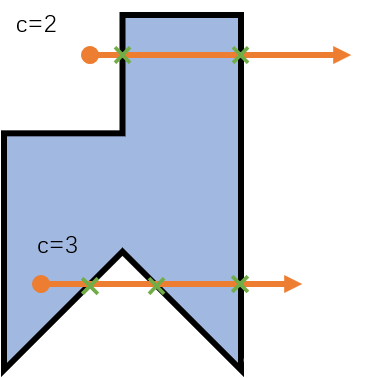通常为了简单起见，射线直接选择为+x方向，也就是向右的方向。

一般优化的思路有：
1大概定义一个多边形的范围，如果点超过这个范围，则无需再判断，直接判断为不在图形内。
2与+x方向射线相交的线段，其y的范围一定要在射线两端。也就是说如果线段在射线的正上方，或者线段在射线的正下方，都不需要再计算交点，因为不可能相交。
3与+x方向射线相交的线段，不可能在射线的左侧。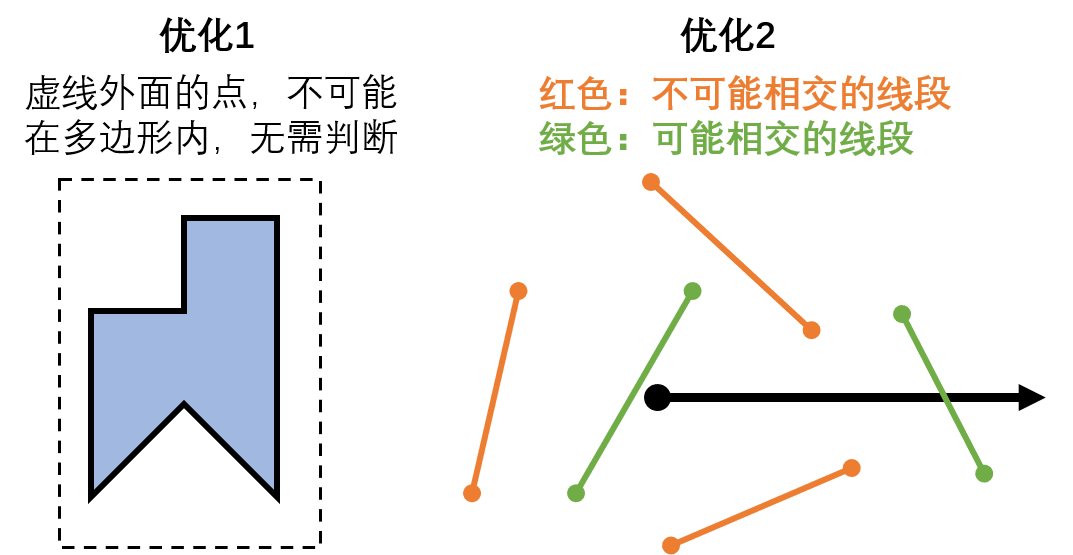这个方法看着很简单，但是还有一些细节需要扣。比如当边线正好也是水平线，怎么计算交点？当点落在边线上，怎么计算交点？当边线正好是水平线，而且点也落在了这个边线上，交叉点算几个？当点的射线正好穿过多边形顶点，会不会得到与两个边相交的bug？

关于这几个问题，我大概解决方法为：设置一个边线点的概念，专门用来判断点是否在边线上。如果点刚好落在水平线的边线上，则不再计算是否相交，直接当做点在边线上处理。交叉点的判断，用一头小于等于，一头小于的方式，避免重复计算。而且因为计算中存在误差，所以对于计算得到的交点xc，需要加一个误差带来防止判断失误。

具体不多说，matlab程序如下：

clear
clc
close all
%多边形定义（连线必须按照首尾相接的顺序）
BD=[0,0;0,-1;1,0;2,-1;2,0;2,1;2,2;1,2;1,1;0,1];%凹多边形
%BD=[0,-1;0,-2;1,-1;2,-2;2,-1;2,0;2,3;-1,3;-1,1;...
%    3,1;3,1.5;0,1.5;0,2.5;1,2.5;1,0;0,0];%凹多边形，而且自相交
%BD=[0,1;-1,0;-1,-1;0,-1;1,-1;1,0];%凸多边形
%要判断的点
[X,Y]=meshgrid(-5:0.1:5,-5:0.1:5);
xy2=[X(:),Y(:)];

[IsInPoly,IsOnBD]=IfInPoly1(BD,xy2);

figure()
hold on
plot(BD(:,1),BD(:,2))
scatter(xy2(:,1),xy2(:,2),24,IsInPoly+2*IsOnBD,'Marker','.')
%scatter(xy2(:,1),xy2(:,2),24,or(IsInPoly,IsOnBD),'Marker','.');%不显示边线

%后置函数
function [IsInPoly,IsOnBD]=IfInPoly1(BD,xy2)
%输出逻辑索引（IsInPoly表示在内部，IsOnBD表示在多边形边界上）
%BD是多边形边界，存在顺序，两列。xy2是点的坐标，两列。
%方法1，射线法
if (BD(1,1)~=BD(end,1)) || (BD(1,2)~=BD(end,2))
BD2=[BD;BD(1,:)];
else %给出的边界已经收尾相接
BD2=BD;
end
%删除边界中相邻重复的点
IsSame=and(BD2(1:end-1,1)==BD2(2:end,1),BD2(1:end-1,2)==BD2(2:end,2));
BD2([IsSame;false],:)=[];
NB=size(BD2,1)-1;
NP=size(xy2,1);

IsInPoly=false(NP,1);%true(NP,1);%false(NP,1);
IsOnBD=false(NP,1);%是否在边线上
%判断整个边线的大概范围
min_X_BD=min(BD(:,1));
max_X_BD=max(BD(:,1));
min_Y_BD=min(BD(:,2));
max_Y_BD=max(BD(:,2));
%做+x方向的射线
for kp=1:NP
%如果这个点的xy超过整个边线的xy，则肯定不在边线内
xy_k=xy2(kp,:);
xp=xy_k(1);
yp=xy_k(2);
if xp<min_X_BD || xp>max_X_BD || yp<min_Y_BD || yp>max_Y_BD
%IsInPoly(kp)=false;
continue
end
%循环每一条边
NCross=0;%初始化交点数量
for kB=1:NB
xB1=BD2(kB,1);xB2=BD2(kB+1,1);
yB1=BD2(kB,2);yB2=BD2(kB+1,2);
%如果在+x方向上相交，在点一定在线的左边
if max([xB1,xB2])<xp
continue %所以点在线右侧的情况无需计算
end
%判断是否在水平的边线上
if yB1==yp && yB2==yp && ( min([xB1,xB2])<=xp && max([xB1,xB2])>=xp)
IsOnBD(kp)=true;%如果点在水平线段上，则证明点在边缘
break %停止循环
end
%判断是否是某个顶点
if (xp==xB1 && yp==yB1) || (xp==xB2 && yp==yB2)
IsOnBD(kp)=true;%如果点是某个边缘顶点，则证明点在边缘
break
end
%如果射线穿过这个边，则y值一定介于这个边的两个y值中间
if (yB1<=yp && yp<yB2) || (yB2<=yp && yp<yB1)
%如果在+x方向上相交，则交点一定在xp的右边
yc=yp;
xc=xB1+(xB2-xB1)/(yB2-yB1)*(yc-yB1);%计算射线与边的交点(xc,yc)
if (xc-xp)>4*eps %由于计算会导致xc有一定的误差，所以保守估算为4*eps
NCross=NCross+1;%证明的确相交，交点+1
end
%再次判断是否在边线上
if abs(xc-xp)<=4*eps && abs(yc-yp)<=4*eps %这里因为也涉及到xc，所以也设置了一个误差带
IsOnBD(kp)=true;%如果交点xc就是点xp，则证明点在线上
break
end
end

end
%根据相交点数的奇偶性判断是否在多边形内
if ~IsOnBD(kp) && mod(NCross,2)
IsInPoly(kp)=true;%如果是奇数，则证明在多边形内
end
end
end


注：这里给出了点在边界内IsInPoly，点在多边形边界上IsOnBD两个结果。如果需要合并，可以用or(IsInPoly,IsOnBD)进行合并结果。

计算结果如下：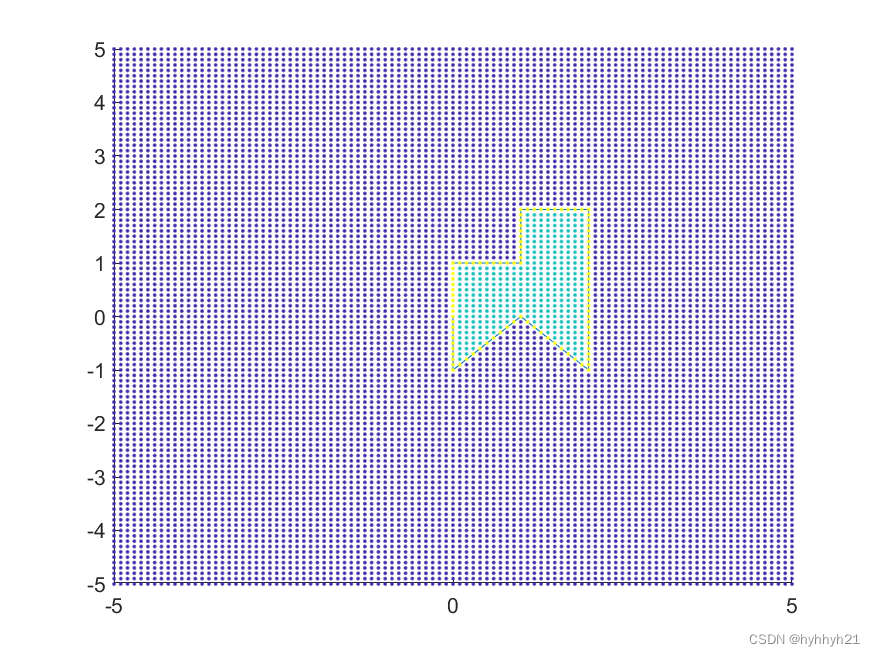# 2 环绕数法（Winding Number）

环绕数法和相交数法类似，都是引出一条射线。但是环绕数不再计算具体交点，而是判断相交的线段，是向上穿过这个射线还是向下穿过这个射线。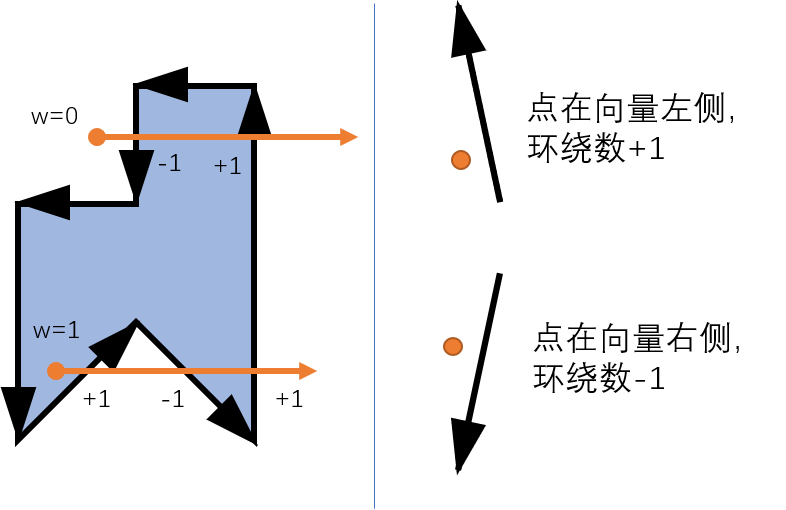举个例子，还是用上一章节的图进行举例子。但是这里的线段都被赋予了方向。我们定义向上穿过射线（点在线段左侧），环绕数+1，向下穿过射线（点在线段右侧），环绕数-1。最终对环绕数相加，等于0说明点在多边形外，不等于0说明点在多边形内。

这个方法的matlab程序如下：

clear
clc
close all
%多边形定义（连线必须按照首尾相接的顺序）
BD=[0,0;0,-1;1,0;2,-1;2,0;2,1;2,2;1,2;1,1;0,1];%凹多边形
%BD=[0,-1;0,-2;1,-1;2,-2;2,-1;2,0;2,3;-1,3;-1,1;...
%    3,1;3,1.5;0,1.5;0,2.5;1,2.5;1,0;0,0];%凹多边形，而且自相交
%BD=[0,1;-1,0;-1,-1;0,-1;1,-1;1,0];%凸多边形
%要判断的点
[X,Y]=meshgrid(-5:0.1:5,-5:0.1:5);
xy2=[X(:),Y(:)];

[IsInPoly,IsOnBD]=IfInPoly2(BD,xy2);

figure()
hold on
plot(BD(:,1),BD(:,2))
scatter(xy2(:,1),xy2(:,2),24,IsInPoly+2*IsOnBD,'Marker','.')
%如果考虑边界情况，则把两个结果合并
%IsInPoly=or(IsInPoly,IsOnBD);

function [IsInPoly,IsOnBD]=IfInPoly2(BD,xy2)
%输出逻辑索引（IsInPoly表示在内部，IsOnBD表示在多边形边界上）
%BD是多边形边界，存在顺序，两列。xy2是点的坐标，两列。
%方法2 winding number 环绕数法
%将边界收尾相接
if (BD(1,1)~=BD(end,1)) || (BD(1,2)~=BD(end,2))
NB=size(BD,1);
BD2=[BD;BD(1,:)];
else %给出的边界已经收尾相接
NB=size(BD,1)-1;
BD2=BD;
end
%删除边界中相邻重复的点
IsSame=and(BD2(1:end-1,1)==BD2(2:end,1),BD2(1:end-1,2)==BD2(2:end,2));
BD2([IsSame;false],:)=[];
NB=size(BD2,1)-1;
NP=size(xy2,1);

IsInPoly=false(NP,1);%true(NP,1);%false(NP,1);
IsOnBD=false(NP,1);%是否在边线上
%判断整个边线的大概范围
min_X_BD=min(BD(:,1));
max_X_BD=max(BD(:,1));
min_Y_BD=min(BD(:,2));
max_Y_BD=max(BD(:,2));
%做+x方向的射线，来判定环绕数
for kp=1:NP
%如果这个点的xy超过整个边线的xy，则肯定不在边线内
xy_k=xy2(kp,:);
xp=xy_k(1);
yp=xy_k(2);
if xp<min_X_BD || xp>max_X_BD || yp<min_Y_BD || yp>max_Y_BD
continue
end
%循环每一条边
NWinding=0;%初始化环绕数
for kB=1:NB
xB1=BD2(kB,1);xB2=BD2(kB+1,1);
yB1=BD2(kB,2);yB2=BD2(kB+1,2);
%如果在+x方向上相交，在点一定在线的左边
if max([xB1,xB2])<xp
continue %所以点在线右侧的情况无需计算
end
%判断是否在水平的边线上
if yB1==yp && yB2==yp && ( min([xB1,xB2])<=xp && max([xB1,xB2])>=xp)
IsOnBD(kp)=true;%如果点在水平线段上，则证明点在边缘
break
end
%判断是否是某个顶点
if (xp==xB1 && yp==yB1) || (xp==xB2 && yp==yB2)
IsOnBD(kp)=true;%如果点是某个边缘顶点，则证明点在边缘
break
end
%如果射线穿过这个边，则y值一定介于这个边的两个y值中间
if (yB1<=yp && yp<yB2) || (yB2<=yp && yp<yB1)
%如果在+x方向上相交，则开始判定点在向量的左边还是右边（向量的左右，不是前面整个几何意义的左右）
if yB2>yB1 %这个边的方向向上
CrossP=(xB2-xB1)*(yp-yB1)-(xp-xB1)*(yB2-yB1);%计算向量差积，判定点在向量左右
if CrossP>0 %点在向量左侧
NWinding=NWinding+1;
end
elseif yB2<yB1 %这个边的方向向下
CrossP=(xB2-xB1)*(yp-yB1)-(xp-xB1)*(yB2-yB1);%计算向量差积，判定点在向量左右
if CrossP<0 %点在向量右侧
NWinding=NWinding-1;
end
else %这个边水平，不计入相交情况
CrossP=-1;
end
%再次判断是否在边线上
if abs(CrossP)<=4*eps %理论上CrossP==0是在边线上，但这里因为涉及到误差计算，所以加了一个4eps。
IsOnBD(kp)=true;
%break
end
end

end
%如果NWinding不是0，则证明在多边形内
if ~IsOnBD(kp) && NWinding
IsInPoly(kp)=true;
end
end

end


这个方法的优势在于，对于自相交图形，环绕数法可以对重叠区域进行区分。比如规定环绕数等于2时，如果用射线法则会判断为点不在图形内，但是环绕数法可以将这个区域区分出来。
（如果规定环绕数为偶数，则点在图形外，环绕数为奇数点在图形内，则环绕数法和射线交点法等价。）

比如下图就展示了一个自相交图形，在同时用交叉点法和环绕数法时，两者对于重叠部分的处理方式。一般的图形不会出现自相交现象，但是如果对于自相交这一部分有比较高的需求的话，可以选择所需要的算法。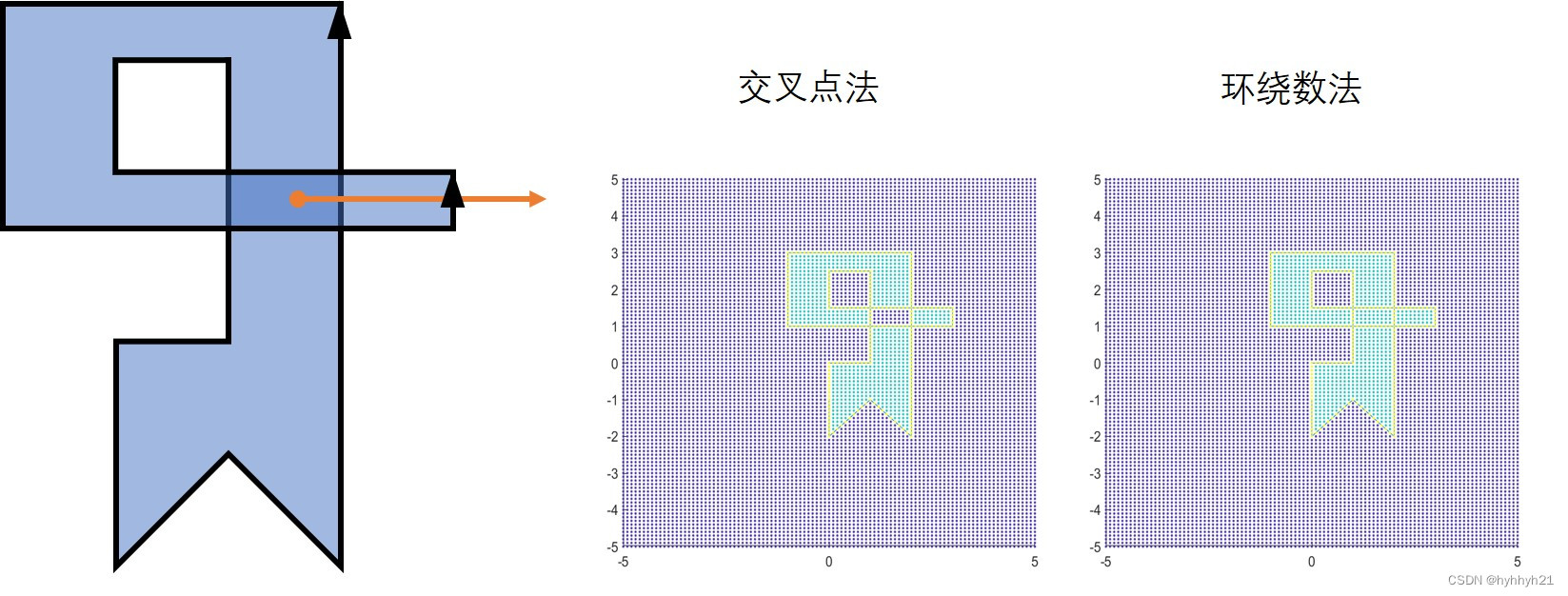当然，环绕数法由于无需计算具体交点，还避免了使用除法。如果对除法也比较敏感的话，可以尝试用环绕数法。

# 3 角度法（转角法）

## 3.1 角度相加法

这个方法比较容易理解，就是如果点在图形内部，则所有射线加起来的角度等于360°。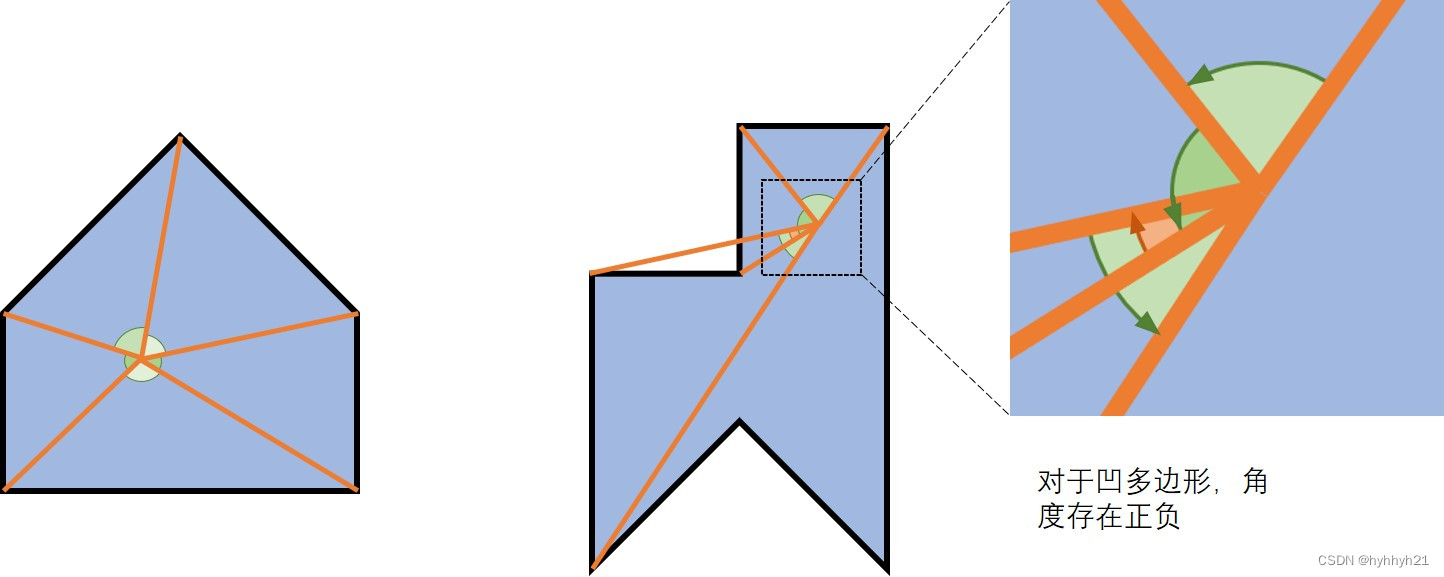但是如果图形是凹多边形，这个方法还需要定义角度的正负。如上图所示，在同一个方向上递增的角度定义为正，在这个方向上突然反向的角度定义为负。具体判定方法，用向量的叉积来判定。

写作公式为：
θ = ∑ ω i = ∑ 1 n − 1 a c o s ( v 1 ⃗ ⋅ v 2 ⃗ ∥ v 1 ⃗ ∥ ∥ v 2 ⃗ ∥ ) ∗ s i g n ( v 1 ⃗ × v 2 ⃗ ) \theta=\sum{\omega_i}=\sum_{1}^{n-1}{acos(\frac{\vec{v_1} \cdot \vec{v_2}}{\left \| \vec{v_1}\right \| \left \| \vec{v_2}\right \|} ) *sign(\vec{v_1}×\vec{v_2})}

这里的v1和v2，是点p到线段两端的两条射线。
这里的基本原理以及结果和环绕数法相同，但是因为涉及到acos的计算，所以通常速度比较慢，后面小节的改进法会讲到怎么加速。

具体程序如下：

clear
clc
close all
%多边形定义（连线必须按照首尾相接的顺序）
BD=[0,0;0,-1;1,0;2,-1;2,0;2,1;2,2;1,2;1,1;0,1];%凹多边形
%BD=[0,-1;0,-2;1,-1;2,-2;2,-1;2,0;2,3;-1,3;-1,1;...
%    3,1;3,1.5;0,1.5;0,2.5;1,2.5;1,0;0,0];%凹多边形，而且自相交
%BD=[0,1;-1,0;-1,-1;0,-1;1,-1;1,0];%凸多边形
%要判断的点
[X,Y]=meshgrid(-5:0.1:5,-5:0.1:5);
xy2=[X(:),Y(:)];
%xy2=[0.5,2];

[IsInPoly,IsOnBD]=IfInPoly3(BD,xy2);

figure()
hold on
plot(BD(:,1),BD(:,2))
scatter(xy2(:,1),xy2(:,2),24,IsInPoly+2*IsOnBD,'Marker','.')

function [IsInPoly,IsOnBD]=IfInPoly3(BD,xy2)
%方法3 计算角度法
%将边界收尾相接
if (BD(1,1)~=BD(end,1)) || (BD(1,2)~=BD(end,2))
NB=size(BD,1);
BD2=[BD;BD(1,:)];
else %给出的边界已经收尾相接
NB=size(BD,1)-1;
BD2=BD;
end
%删除边界中相邻重复的点
IsSame=and(BD2(1:end-1,1)==BD2(2:end,1),BD2(1:end-1,2)==BD2(2:end,2));
BD2([IsSame;false],:)=[];
NB=size(BD2,1)-1;
NP=size(xy2,1);

IsInPoly=false(NP,1);%true(NP,1);%false(NP,1);
IsOnBD=false(NP,1);%是否在边线上
%判断整个边线的大概范围
min_X_BD=min(BD(:,1));
max_X_BD=max(BD(:,1));
min_Y_BD=min(BD(:,2));
max_Y_BD=max(BD(:,2));
%做+x方向的射线，来判定环绕数
for kp=1:NP
%如果这个点的xy超过整个边线的xy，则肯定不在边线内
xy_k=xy2(kp,:);
xp=xy_k(1);
yp=xy_k(2);
if xp<min_X_BD || xp>max_X_BD || yp<min_Y_BD || yp>max_Y_BD
continue
end
%判断是否是某个顶点
if any(and(xp==BD2(:,1),yp==BD2(:,2)))
%IsOnBD(kp)=true;%如果点是某个边缘顶点，则证明点在边缘
IsOnBD(kp)=true;
continue
end
%循环每一条边
AngleSum=0;%初始化环绕数
for kB=1:NB

xB1=BD2(kB,1);xB2=BD2(kB+1,1);
yB1=BD2(kB,2);yB2=BD2(kB+1,2);
v1=[xB1-xp,yB1-yp];v2=[xB2-xp,yB2-yp];
CosAngle=dot(v1,v2)/norm(v1)/norm(v2);
if abs(CosAngle+1)<4*eps
IsOnBD(kp)=true;
break
end
Sign=sign(det([v1(1),v2(1);v1(2),v2(2)]));
AngleSum=acos(CosAngle)*Sign+AngleSum;
%AngleList(kB)=acos(CosAngle)*Sign/pi*180;
end
if abs(AngleSum)/2/pi>(1-1e-5)
IsInPoly(kp)=true;
end
end
IsInPoly=and(IsInPoly,~IsOnBD);%对于那些又在边线上又边线内的，判定为在边线上。

end


## 3.2 改进角度法（matlab自带inpolygon函数方法）

matlab自带inpolygon()函数来判定点是否在多边形内。

这里不再具体的计算角度，而是利用射线x和y的关系，进行大致判断。首先将点与边线上各个顶点相连，组成一组射线。将射线方向分为右上↗、左上↖、左下↙、右下↘这四个象限，分别赋予0、1、2、3这4个数字。然后根据这几个数字的变化，判断最终角度。

具体的方法可以参见matlab函数inpolygon的具体代码，或者看论文The point in polygon problem for arbitrary polygons，这里我就不再献丑重新自己编了。

具体使用方法如下

clear
clc
close all
%多边形定义（连线必须按照首尾相接的顺序）
BD=[0,0;0,-1;1,0;2,-1;2,0;2,1;2,2;1,2;1,1;0,1];%凹多边形
%BD=[0,-1;0,-2;1,-1;2,-2;2,-1;2,0;2,3;-1,3;-1,1;...
%    3,1;3,1.5;0,1.5;0,2.5;1,2.5;1,0;0,0];%凹多边形，而且自相交
%BD=[0,1;-1,0;-1,-1;0,-1;1,-1;1,0];%凸多边形
%要判断的点
[X,Y]=meshgrid(-5:0.1:5,-5:0.1:5);
xy2=[X(:),Y(:)];

%方法6 matlab自带方法
[IsInPoly,IsOnBD]=inpolygon(xy2(:,1),xy2(:,2),BD(:,1),BD(:,2));

figure()
hold on
plot(BD(:,1),BD(:,2))
scatter(xy2(:,1),xy2(:,2),24,IsInPoly+2*IsOnBD,'Marker','.')


我用的是2019b版本的，有几个边线点没有识别出来，估计是没有引入误差的结果。但是运行速度很快。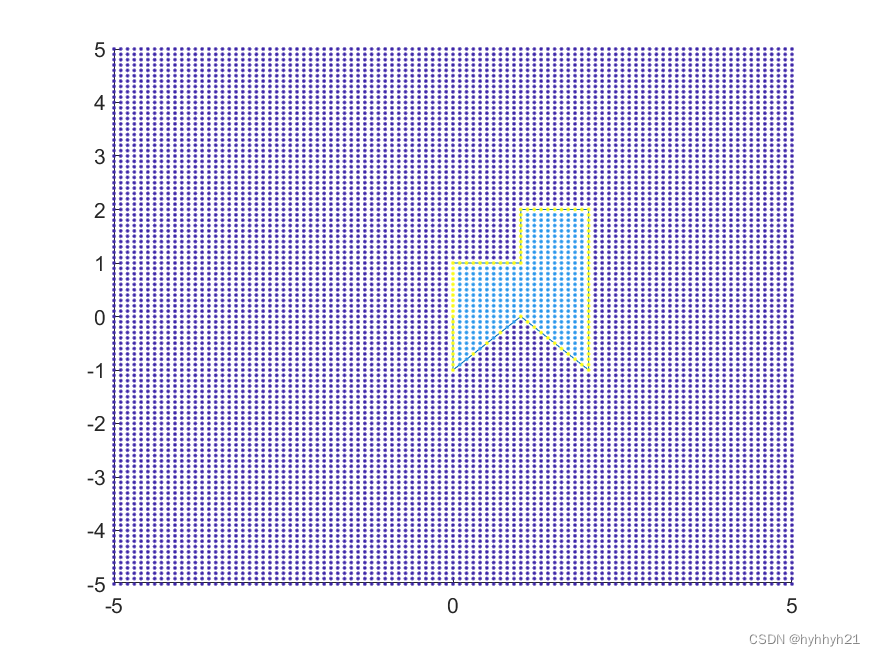# 4 叉积法（只适用于凸多边形）

叉积法的思路来源于角度法。对于凸多边形来说，点在多边形内的话，将该点与多边形各个顶点相连组成一系列向量，这些向量的夹角一定是锐角。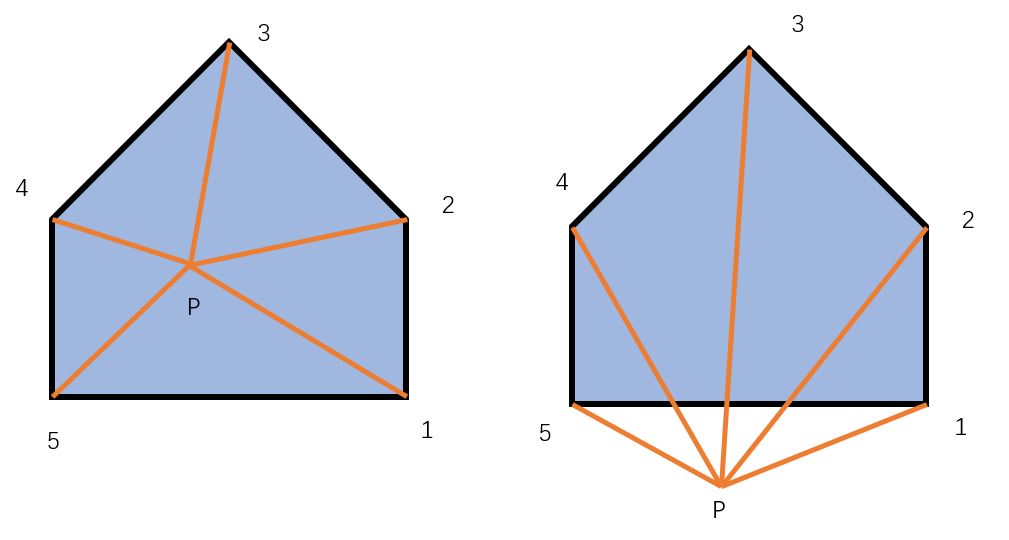比如上左图，P1和P2叉积是正的，P2和P3叉积也为正，同理P3和P4，P4和P5，P5和P1都为正。但是对于右图，P5和P1的叉积就是负值，说明点在多边形外。

代码如下：

clear
clc
close all
%多边形定义（连线必须按照首尾相接的顺序）
%BD=[0,0;0,-1;1,0;2,-1;2,0;2,1;2,2;1,2;1,1;0,1];%凹多边形
%BD=[0,-1;0,-2;1,-1;2,-2;2,-1;2,0;2,3;-1,3;-1,1;...
%    3,1;3,1.5;0,1.5;0,2.5;1,2.5;1,0;0,0];%凹多边形，而且自相交
BD=[0,1;-1,0;-1,-1;0,-1;1,-1;1,0];%凸多边形
%要判断的点
[X,Y]=meshgrid(-5:0.1:5,-5:0.1:5);
xy2=[X(:),Y(:)];
%xy2=[0.8,0.8];

IsInPoly=IfInPoly4(BD,xy2);

figure()
hold on
plot(BD(:,1),BD(:,2))
%scatter(xy2(:,1),xy2(:,2),24,IsInPoly+2*IsOnBD,'Marker','.')
scatter(xy2(:,1),xy2(:,2),24,IsInPoly,'Marker','.')

function IsInPoly=IfInPoly4(BD,xy2)
%方法4 计算叉积法
%将边界收尾相接
if (BD(1,1)~=BD(end,1)) || (BD(1,2)~=BD(end,2))
NB=size(BD,1);
BD2=[BD;BD(1,:)];
else %给出的边界已经收尾相接
NB=size(BD,1)-1;
BD2=BD;
end
%删除边界中相邻重复的点
IsSame=and(BD2(1:end-1,1)==BD2(2:end,1),BD2(1:end-1,2)==BD2(2:end,2));
BD2([IsSame;false],:)=[];
NB=size(BD2,1)-1;
NP=size(xy2,1);

IsInPoly=false(NP,1);%true(NP,1);%false(NP,1);
IsOnBD=false(NP,1);%是否在边线上
%判断整个边线的大概范围
min_X_BD=min(BD(:,1));
max_X_BD=max(BD(:,1));
min_Y_BD=min(BD(:,2));
max_Y_BD=max(BD(:,2));
%做+x方向的射线，来判定环绕数
for kp=1:NP
%如果这个点的xy超过整个边线的xy，则肯定不在边线内
xy_k=xy2(kp,:);
xp=xy_k(1);
yp=xy_k(2);
if xp<min_X_BD || xp>max_X_BD || yp<min_Y_BD || yp>max_Y_BD
continue
end
%判断是否是某个顶点
if any(and(xp==BD2(:,1),yp==BD2(:,2)))
%IsOnBD(kp)=true;%如果点是某个边缘顶点，则证明点在边缘
IsInPoly(kp)=true;
continue
end
%循环每一条边
ArrowDir=zeros(NB,1);%初始化叉积方向
for kB=1:NB
xB1=BD2(kB,1);xB2=BD2(kB+1,1);
yB1=BD2(kB,2);yB2=BD2(kB+1,2);
v1=[xB1-xp,yB1-yp,0];v2=[xB2-xp,yB2-yp,0];
CrossV=cross(v1,v2);
ArrowDir(kB)=CrossV(3);
end
%根据最大角度和最小角度之差
if all(ArrowDir>=0) || all(ArrowDir<=0)
IsInPoly(kp)=true;
end
end

end


结果如下图：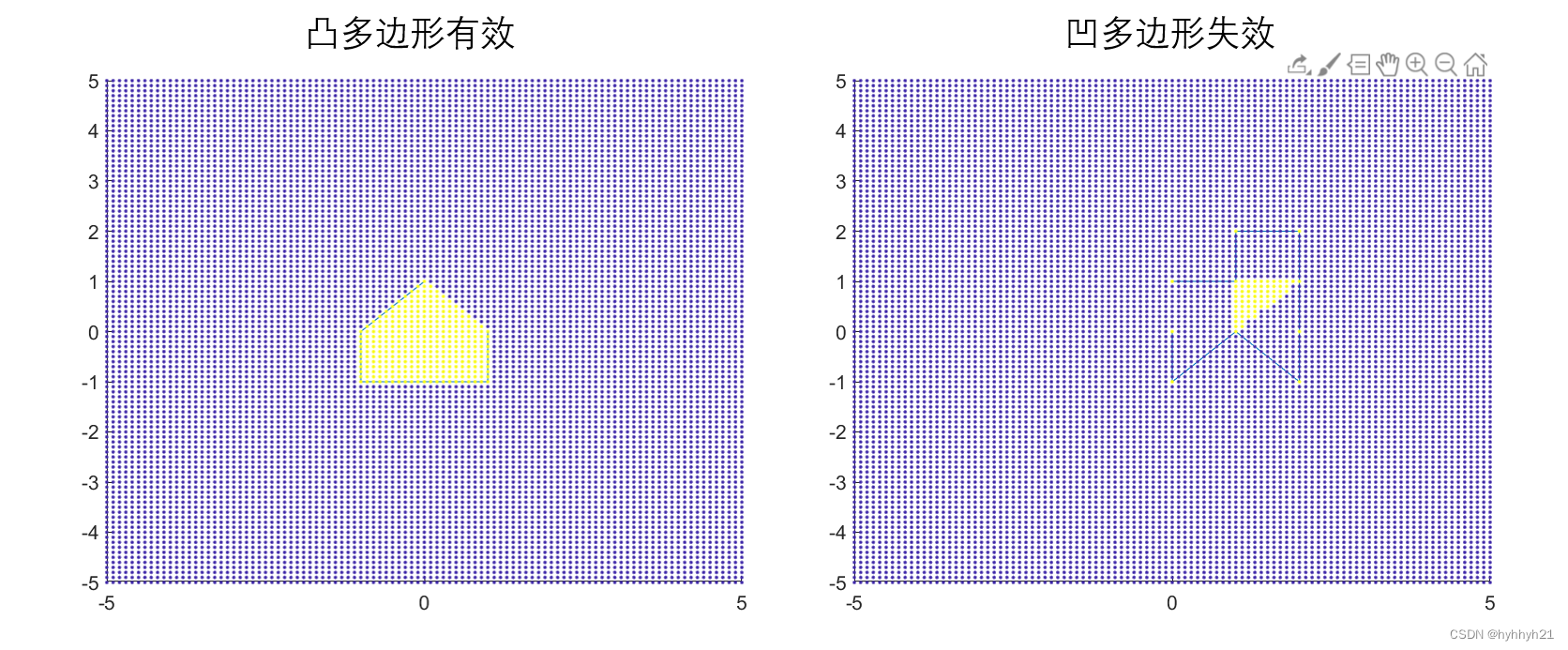可以看到对于凸多边形，这个算法还是没问题的。但是对于凹多边形，这个方法就会产生错误的结果。所以用这个方法一定要注意是否为凸多边形。

# 5 网格法

网格法顾名思义，就是由于多边形形状太过于复杂，无法简单的进行判断。那么就可以先给多边形内部画上许多个网格，然后依次判断点是否在网格内就可以。

矩形网格的优点在于判断速度快，但是对于斜边的判断比较复杂。有可能导致矩形的一部分在多边形内，一部分在多边形外。

这里用三角形网格进行简单的判断。首先就是划分三角形，这里我用的是matlab自带的delaunayTriangulation()函数。
判断点是否在三角形内，可以用两个向量解二元二次方程得到。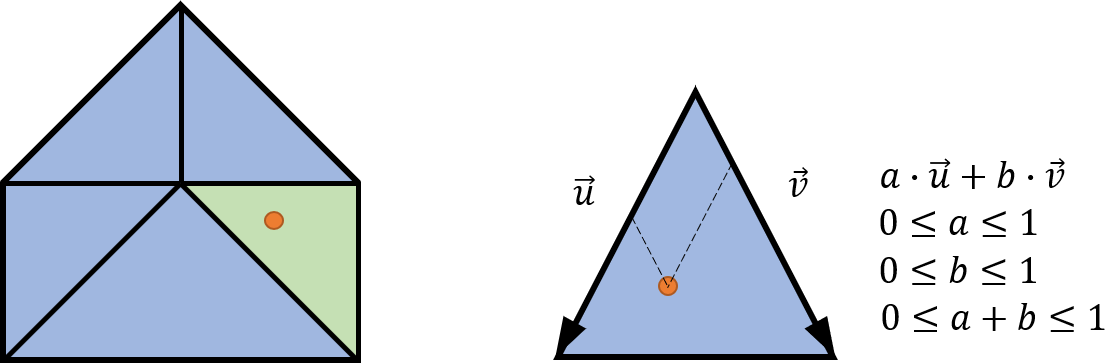这个点的位置可以用au+bv来确定，如果满足a、b、a+b都在0到1之间，则该点肯定在三角形内。

具体程序如下：

clear
clc
close all
%多边形定义（连线必须按照首尾相接的顺序）
BD=[0,0;0,-1;1,0;2,-1;2,0;2,1;2,2;1,2;1,1;0,1];%凹多边形
%BD=[0,-1;0,-2;1,-1;2,-2;2,-1;2,0;2,3;-1,3;-1,1;...
%    3,1;3,1.5;0,1.5;0,2.5;1,2.5;1,0;0,0];%凹多边形，而且自相交
%BD=[0,1;-1,0;-1,-1;0,-1;1,-1;1,0];%凸多边形
%要判断的点
[X,Y]=meshgrid(-5:0.1:5,-5:0.1:5);
xy2=[X(:),Y(:)];

IsInPoly=IfInPoly5(BD,xy2);

figure()
hold on
plot(BD(:,1),BD(:,2))
scatter(xy2(:,1),xy2(:,2),24,IsInPoly,'Marker','.')

function IsInPoly=IfInPoly5(BD,xy2)
%方法5 三角形网格剖分法
%如果边界收尾相接，则取消
if (BD(1,1)==BD(end,1)) && (BD(1,2)==BD(end,2))
BD2=BD(1:end-1,:);
else %给出的边界已经收尾相接
BD2=BD;
end
%删除边界中相邻重复的点
IsSame=and(BD2(1:end-1,1)==BD2(2:end,1),BD2(1:end-1,2)==BD2(2:end,2));
BD2([IsSame;false],:)=[];
NB=size(BD2,1);
NP=size(xy2,1);

IsInPoly=false(NP,1);%true(NP,1);%false(NP,1);
%IsOnBD=false(NP,1);%是否在边线上
%判断整个边线的大概范围
min_X_BD=min(BD2(:,1));
max_X_BD=max(BD2(:,1));
min_Y_BD=min(BD2(:,2));
max_Y_BD=max(BD2(:,2));

%按照边缘三角剖分
C=(1:NB)';
C=[C,[(2:NB)';1]];
DT = delaunayTriangulation(BD2,C);
%剔除落在外面的三角形
IO = isInterior(DT);
CL=DT.ConnectivityList;
CL(~IO,:)=[];
BD2=DT.Points;%点有可能会被网格划分所更新，所以这里重新加载一下
NB=size(BD2,1);

BDx=BD2(:,1);
BDy=BD2(:,2);
%triplot(CL,BDx,BDy)
%得到每个三角形的坐标
TRI_X=BDx(CL);
TRI_Y=BDy(CL);
NT=size(CL,1);
%计算每个三角形的范围
max_TRI_X=max(TRI_X,[],2);
min_TRI_X=min(TRI_X,[],2);
max_TRI_Y=max(TRI_Y,[],2);
min_TRI_Y=min(TRI_Y,[],2);
%计算每个三角形的向量
V1_Sum=[TRI_X(:,2)-TRI_X(:,1),TRI_Y(:,2)-TRI_Y(:,1)];
V2_Sum=[TRI_X(:,3)-TRI_X(:,1),TRI_Y(:,3)-TRI_Y(:,1)];
%开始循环判断
for kp=1:NP
%如果这个点的xy超过整个边线的xy，则肯定不在边线内
xy_k=xy2(kp,:);
xp=xy_k(1);
yp=xy_k(2);
if xp<min_X_BD || xp>max_X_BD || yp<min_Y_BD || yp>max_Y_BD
continue
end
%判断是否是某个顶点
if any(and(xp==BD2(:,1),yp==BD2(:,2)))
%IsOnBD(kp)=true;%如果点是某个边缘顶点，则证明点在边缘
IsInPoly(kp)=true;
continue
end
%循环每一三角形
for kT=1:NT
if xp<min_TRI_X(kT) || xp>max_TRI_X(kT) || yp<min_TRI_Y(kT) || yp>max_TRI_Y(kT)
continue %如果超出三角形范围，则直接跳过
end
%计算是否在三角形内
V1=V1_Sum(kT,:);
V2=V2_Sum(kT,:);
A=[V1',V2'];
B=[xp-TRI_X(kT,1);yp-TRI_Y(kT,1)];
u12=A\B;
if max(u12)<=1 && min(u12)>=0 && sum(u12)<=1
IsInPoly(kp)=true;
break
end
end
end
end


网格划分结果以及最终图案如下：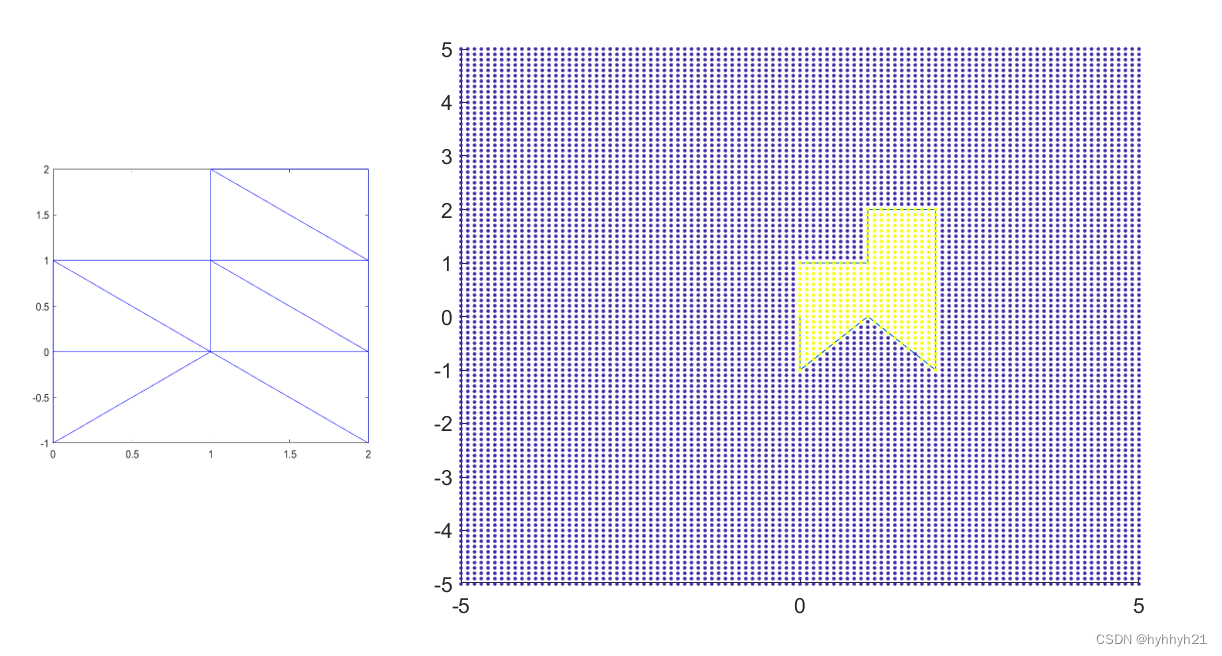# 6 二分法（O(logn)算法）

如果多边形的边数非常多，则用之前的方法计算，复杂度都是O(n)级别的，要循环的边数和图形成正比。（虽然感觉如果图形比较简单，优化比较好的话，那么射线法和环绕数法中，很多边都循环不到，应该也不会太慢）。

那么二分法的思路就是逐步缩小范围，找到点所在的区域。算法图示如下：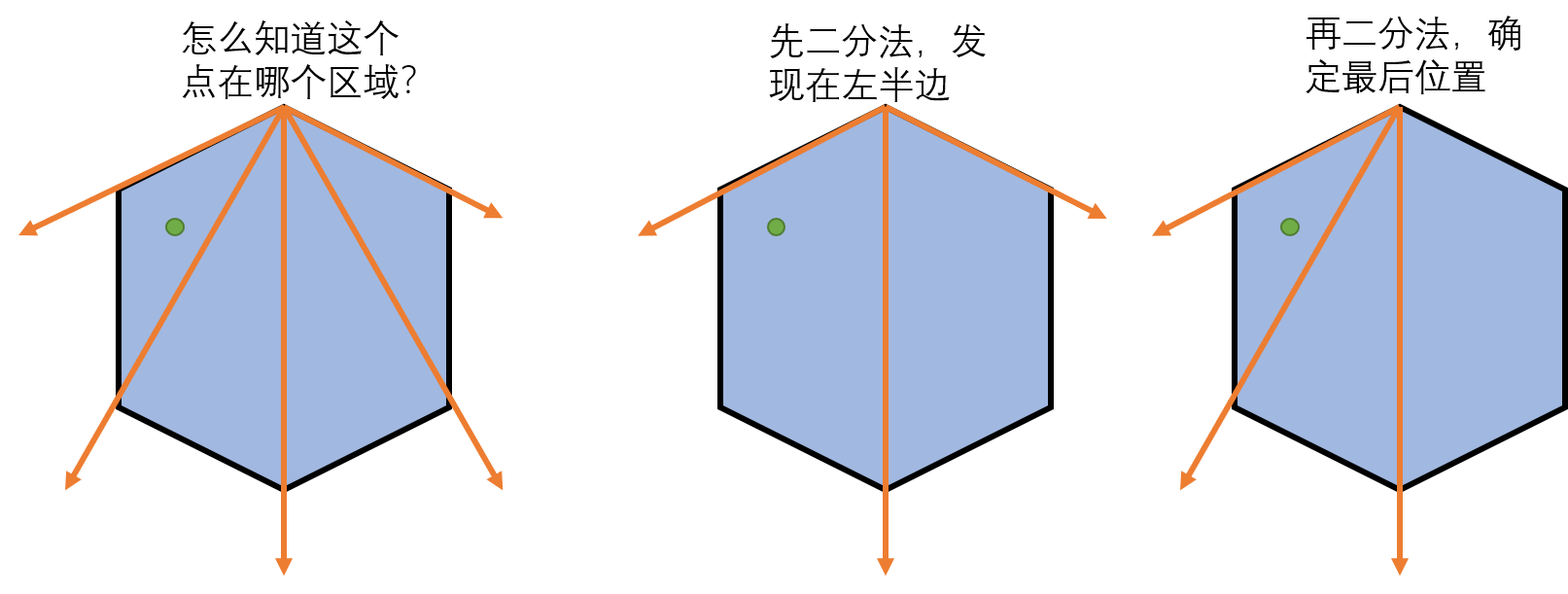先任取一个顶点，然后对其它顶点做射线。如果点在多边形内，那么点一定在这个夹角范围之内。然后通过逐步缩小范围，确定点的最终位置。
之后，我们就可以用前面第5章判断点在三角形内的方法，判断这个点是否在这个三角形内。

具体程序如下：

clear
clc
close all
%多边形定义（连线必须按照首尾相接的顺序）
%BD=[0,0;0,-1;1,0;2,-1;2,0;2,1;2,2;1,2;1,1;0,1];%凹多边形
%BD=[0,-1;0,-2;1,-1;2,-2;2,-1;2,0;2,3;-1,3;-1,1;...
%    3,1;3,1.5;0,1.5;0,2.5;1,2.5;1,0;0,0];%凹多边形，而且自相交
BD=[0,1;-1,0;-1,-1;0,-1;1,-1;1,0];%凸多边形
BD=[2.5*cos(0.01:0.01:2*pi)',2*sin(0.01:0.01:2*pi)'];
%要判断的点
[X,Y]=meshgrid(-5:0.1:5,-5:0.1:5);
xy2=[X(:),Y(:)];

IsInPoly=IfInPoly7(BD,xy2);

figure()
hold on
plot(BD(:,1),BD(:,2))
scatter(xy2(:,1),xy2(:,2),24,IsInPoly,'Marker','.')

function IsInPoly=IfInPoly7(BD,xy2)
%方法7 二分法
NP=size(xy2,1);
BD2=BD;
%1初始化输入
%删除边界中相邻重复的点
IsSame=and(BD2(1:end-1,1)==BD2(2:end,1),BD2(1:end-1,2)==BD2(2:end,2));
BD2([IsSame;false],:)=[];
BD=BD2;
%将边界收尾相接
if (BD(1,1)~=BD(end,1)) || (BD(1,2)~=BD(end,2))
BD2=[BD;BD(1,:)];
else %给出的边界已经收尾相接
BD2=BD;
end
%删除三点共线情况的点
Is3Line=false(size(BD2,1)-1,1);
for k=2:size(BD2,1)-1
if det([ BD2(k-1,:)-BD2(k,:) ; BD2(k+1,:)-BD2(k,:) ])==0
Is3Line(k)=true;%如果三点共线，则叉积等于0
end
end
BD2([Is3Line;true],:)=[];%删除三点共线的那些点
if det([ BD2(end,:)-BD2(1,:) ; BD2(2,:)-BD2(1,:) ])==0
BD2(1,:)=[];%刚才循环没有判断第一个点，重新判断一下
end
NB=size(BD2,1);
%如果边的方向是顺时针方向，则变成逆时针排序方向
xy0=BD2(1,:);
v1_t=BD2(2,:)-xy0;
v2_t=BD2(NB,:)-xy0;
if det([v1_t;v2_t])<eps
BD2=flipud(BD2);%小于0，说明给出的点是顺时针排序的
end

IsInPoly=false(NP,1);
IsOnBD=false(NP,1);%是否在边线上
%判断整个边线的大概范围
min_X_BD=min(BD(:,1));
max_X_BD=max(BD(:,1));
min_Y_BD=min(BD(:,2));
max_Y_BD=max(BD(:,2));
%2确定起始边和终止边
%以第一个点作为射线基准，计算出所有点的射线
vSum=BD2(2:end,:)-ones(NB-1,1)*BD2(1,:);
Nv=size(vSum,1);
for kp=1:NP
%3优化，减少计算数量
%如果这个点的xy超过整个边线的xy，则肯定不在边线内
xy_k=xy2(kp,:);
xp=xy_k(1);
yp=xy_k(2);
if xp<min_X_BD || xp>max_X_BD || yp<min_Y_BD || yp>max_Y_BD
continue
end
%判断是否是某个顶点
if any(and(xp==BD2(:,1),yp==BD2(:,2)))
%IsOnBD(kp)=true;%如果点是某个边缘顶点，则证明点在边缘
IsInPoly(kp)=true;
continue
end
%判断是否在两端射线范围内
v0_t=xy_k-BD2(1,:);
v1_t=vSum(1,:);
v2_t=vSum(Nv,:);
if det([v1_t;v0_t])>=0 && det([v0_t;v2_t])>=0
%如果在两个夹角范围内，则开始后续的二分法循环
n1=1;
n2=Nv;
else
continue
end
%4开始用二分法判断是否在区间内
while n2-n1>1 %当区间大于1的时候，继续二分
v1_t=vSum(n1,:);
v2_t=vSum(n2,:);
n3=fix((n1+n2)/2);
v3_t=vSum(n3,:);

if det([v1_t;v0_t])>=0 && det([v0_t;v3_t])>=0
%在第一个区间
n1=n1;
n2=n3;
else %在第二个区间
n1=n3;
n2=n2;
end
end
%5二分法结束后，确定该点是否在两个向量所围成的三角形中,
v1_t=vSum(n1,:);
v2_t=vSum(n2,:);
A=[v1_t',v2_t'];
B=[xp-BD2(1,1);yp-BD2(1,2)];
u12=A\B;
if max(u12)<=1 && min(u12)>=0 && sum(u12)<=1
IsInPoly(kp)=true;%如果点在这个三角形内，则证明点在多边形内
end
end
end


最终结果如下：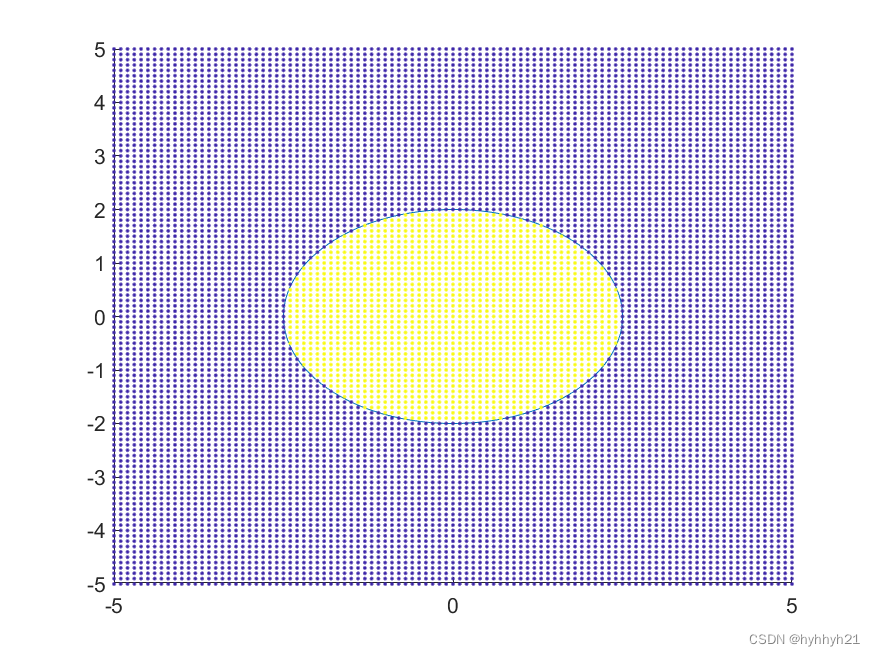# 7 多个区域之间

如果涉及到多个区域之间的相互关系，可以用逻辑运算符的交并差操作，来逐步划分出所要选择的区域。

比如下图，就展示了两个图形相交后，各个区域提取的结果：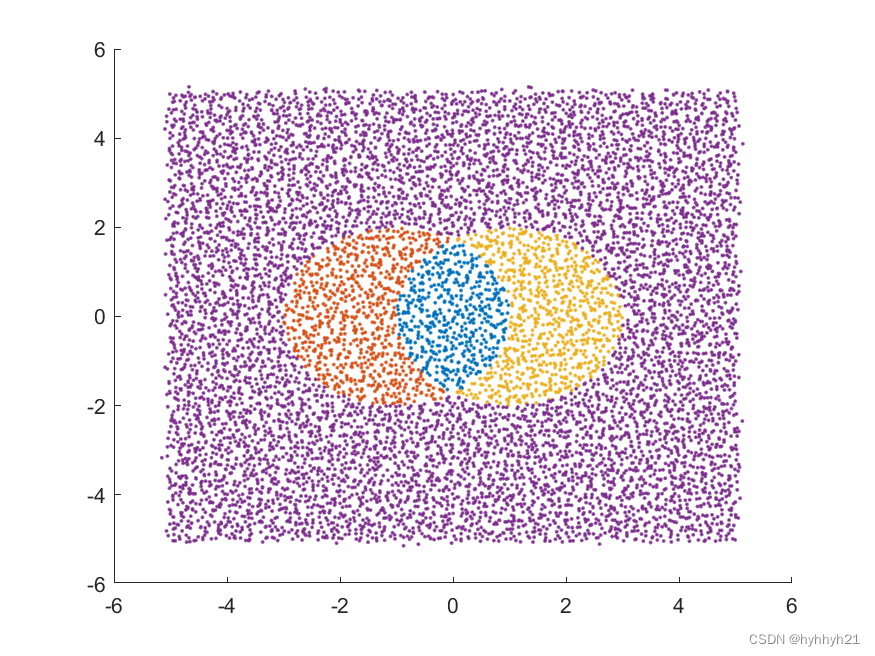代码如下：

%多边形定义（连线必须按照首尾相接的顺序）
BD1=[2*cos(0.01:0.01:2*pi)'-1,2*sin(0.01:0.01:2*pi)'];
BD2=[2*cos(0.01:0.01:2*pi)'+1,2*sin(0.01:0.01:2*pi)'];
%要判断的点
[X,Y]=meshgrid(-5:0.1:5,-5:0.1:5);
X=X+randn(size(X))*0.05;
Y=Y+randn(size(Y))*0.05;
xy2=[X(:),Y(:)];

%判断点是否在图形内
IsInPoly1=inpolygon(xy2(:,1),xy2(:,2),BD1(:,1),BD1(:,2));
IsInPoly2=inpolygon(xy2(:,1),xy2(:,2),BD2(:,1),BD2(:,2));

%交集
Area1=and(IsInPoly1,IsInPoly2);
Area2=and(IsInPoly1,~IsInPoly2);
Area3=and(~IsInPoly1,IsInPoly2);
Area4=and(~IsInPoly1,~IsInPoly2);

figure()
hold on
scatter(xy2(Area1,1),xy2(Area1,2),24,1*ones(sum(Area1),1),'Marker','.')
scatter(xy2(Area2,1),xy2(Area2,2),24,2*ones(sum(Area2),1),'Marker','.')
scatter(xy2(Area3,1),xy2(Area3,2),24,3*ones(sum(Area3),1),'Marker','.')
scatter(xy2(Area4,1),xy2(Area4,2),24,4*ones(sum(Area4),1),'Marker','.')
colormap(lines(4))

展开全文matlab 算法
• 这是一个简单的打字稿应用程序，用于从监视多边形验证器节点。 它检查： 您的签名密钥上的ETH数量 Heimdall块高度与外部API Bor Block Heigh与外部API 您的签名位于最新的Heimdall街区 将来，它还应该检查： 您...
• 24.3正多边形和圆 即:习点病份你解:举一反三 课时训练基础达标 知能提升作业 点击进入相应模块 目录章首未页 础梳理,预习点睛 基础梳理预习点睛 ... 预 点日标提握正多边形的相关概念,会依据圆的性质证明 时训练基础达
• users_auth 基于django-angular-token-auth（ ）JavaScript
• 标签 使用Python进行图像多边形注释 ... GUI自定义（预定义标签/标志，自动保存，标签验证等）。 （ ） 导出VOC格式的数据集以进行语义/实例分割。 （，） 导出COCO格式的数据集以进行实例细分。 （） 要求 Ubunt
• 考虑轮胎接地摩擦的非线性特性以及胎面与路面相互作用导致的磨损,建立了基于LuGre摩擦模型的胎面侧向自激振动动力学模型,分析轮胎多边形磨损的产生条件和磨损边数的计算公式,并通过数值仿真进行了验证。结果表明,...
• 为了解决大规模复杂多边形数据合并运算效率问题, 减少在空间数据库中检索多边形时的磁盘读取次数, 针对多核环境下简单要素类多边形合并的特点, 利用Hilbert曲线划分方法对简单要素的多边形进行空间数据划分, 利用...
• CityGML验证多边形 根据验证 CityGML 多边形 用法 var citygmlPolygons = require ( "citygml-polygons" ) ; var citygmlValidatePolygon = require ( "citygml-validate-polygon" ) ; var xml = "..." ; // Some ...JavaScript
• 高德地图实现多边形框选，选中展示地图中坐标点。实现坐标点框选。范围内坐标点选择。包括矩形，圆形，和任意多边形。。。需要修改key为自己注册的开发key
• 上图显示了一个具有14条边的凹多边形。我们要判断红色点是否在多边形内。 解决方案是将测试点的Y坐标与多边形的每一个点进行比较，我们会得到一个测试点所在的行与多边形边的交点的列表。在这个例子中有8条边与测试...算法
• 在Ghosh的斜率图法(slope diagram)基础之上，对凹—凹这种情况进行了重点研究分析,提出了一种更加高效的求取NFP的改进算法。新的改进算法改进了边的遍历方式，简化了...仿真实例验证了所提方法的正确性和有效性。分段
• 确定点是在简单多边形内部还是外部的一种简单方法是测试从该点开始并沿任何固定方向行进的射线与多边形边缘相交的次数。 如果所讨论的点不在多边形的边界上，则该点在外部时为偶数，在内部时为奇数。 该算法基于一...Python
• 文中提出了一种基于GPU的栅格化多边形相交面积算法GPURAS，在此基础上，分别采用蒙特卡罗方法和遮挡查询技术进一步提出GPURASMC算法和GPURASQ算法，并证明了上述算法的正确性。实验对简单多边形、任意复杂多边形及...
• 什么是凸多边形和凹多边形？ 凸多边形：每个内角都是锐角或钝角，没有大于180度的内角(例如，三角形、正方形)。 凹多边形：至少有一个大于180度的内角(例如，五角星)。 注：大于180度的角又被称为优角 如上...
• 某个课程作业，花了6小时复现的...数据源是一个随机矩阵，三角网络的计算结果与matlab内建函数做了对比，证明没算错。具体实现上没严格按照资料上来，有一些自己的想法（其实主要是找的资料不详细也懒得深入调研了）
• 探讨了平面多边形的Voronoi图的性质，提出了一种新的求解平面多边形的Voronoi图的算法，其核心思想是单独考虑每个Voronoi图对象，分开计算其分治区，然后再构成一个具有拓扑关系的Voronoi图．这种计算方法较其他现有...
• 使用 django 和 gmap 绘制和验证多边形的简单示例 安装 安装 Python 2.7.3 和 PIP，要查看有关如何安装所有内容以使 django 正常工作的完整详细信息，请参见： 如果您已经使用 python 和 pip： pip 安装 Django==...JavaScript
• 对“简单多边形三角形化图S是可以3-色”的定理证明中用到的关键定理：“简单多边形三角形化图S的对偶图T是一棵树”作了十分简化的证明，从而简化了3-色问题及Art Gallery问题Watchman定理的证明
• 多边形最小外接矩形算法 程鹏飞 题目 给出求一个多边形最小外接矩形的算法并证明求得的是最小外接矩形. 最小外接矩形英文简称为SMBR(smallest minimum bounding rectangle,它和MBR(minimum bounding rectangle)不...
• 定理 1 在所有定边长的 NNN 边形中，圆内接凸...然后若多边形的各点不全共圆，一定能按如下方法找到面积更大的多边形方法如下： 设多边形上的点组成的点集为 {G1、G2...GN}\{G_1、G_2 ... G_N\}{G1​、G2​...GN...数学
• 来源： ... 今天是5月14日，我们按照规定，给出几道答案等于14的题目。...请您自行验证一下正六边形内划分出三角形的方法共有多少种（n取6时）。上面是对正多边形进行的讨论。其实对凸多边形都是成立的。...